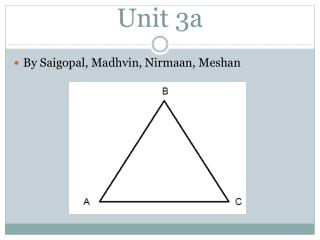DownloadDownload PresentationUnit 3a

# Unit 3a

Télécharger la présentation## Unit 3a

- - - - - - - - - - - - - - - - - - - - - - - - - - - E N D - - - - - - - - - - - - - - - - - - - - - - - - - - -
##### Presentation Transcript

1. Unit 3a By Saigopal, Madhvin, Nirmaan, Meshan
3. Terminal Arm Diagrams Two rays are needed in order to create an angle, both these rays are called the Initial arm and the Terminal Arm. The Initial arm and Terminal arm are placed along the positive x-axis. Terminal arm “Rotates Counter-Clockwise Initial arm “Stays Constant”
4. The Cast Rule First quadrant: “A” signifies that all the primary trig ratios are positive. Second quadrant: “S” signifies that only sine is positive and cosine and tangent are negative. Third quadrant: “T” signifies that only tangent is positive and sine and cosine are negative. Fourth quadrant: “C” signifies that only cosine is positive and sine and tangent are negative.
5. The Cast Rule (cont) Always remember that the CAST Rule is a quick way to see which quadrants the trig ratio is positive.
6. The Cast Rule Example 1 Determine the value of , if a) sin = 0.9063 b) tan = -0.8098
7. Reciprocal Functions and Negative Angles Primary Trig Ratios Reciprocal Functions Sine: Cosecant: Cosine: Secant: Tangent: Cotangent:
8. Reciprocal Functions and Negative Angles Since we now use the reciprocal form the ratio names change as well* Sine to Cosecant: (sin-csc) Cosine to Secant: (cos-sec) Tangent to Cotangent: (tan-cot)
9. Reciprocal Functions and Negative Angles The Reciprocal Functions would be either positive or negative in the same quadrants as their primary functions are. S A Sine Cosecant All T C Tangent Cotangent Cosine Secant
10. Reciprocal Functions and Negative Angles Negative Angles - Positive angles travel counter clock wise - Negative angles travel clockwise
11. Reciprocal Functions and Negative Angles Coterminal Angles: - Angles sharing the same terminal arm - Found by adding or subtracting 360º
12. Example Solve Sec53= Determine the exact value: cos-30
13. Special Triangles
14. Steps of Determining Exact Trig Ratios Make terminal arm in appropriate quadrant Label the related angle. The value should either be 30, 60, or 45 Solve for appropriate trigonometric ratio(s) Use CAST rule to check
15. The Special Angle Example Example 2 Determine the exact value of the following a) cos315= b) sin120=
16. The Ambiguous Case This occurs when you are exposed to the following: Two sides and an angle opposite one of those sides. B would be thefirst piece of information you would solve for in the triangle to the right. However, due to the fact that the sine ratios of supplementary angles are equal, it is possible to have an outcome of more than one triangle.
17. The Ambiguous Case There are two cases you must take in to consideration within the unit. If A is obtuse there can be a total of two possible results: 0 or 1 triangles exist. If A is acute then there can be three outcomes: either 0, 1, or 2 triangles exist.
18. The Ambiguous Case We know that the value of y will always be smaller than the value of r in each of the quadrants. As a result, the value of y/r will always be between -1 and +1. The value of sinθ will always be between -1 and 1.
19. Steps to Follow 1. Draw out the triangle 2. See if the angle is acute or obtuse 3. In this case it is acute meaning that there can be 0, 1, 2 triangle possibilities 4. Find out the angle B using sine law. 5. In this situation there can be 3 possibilities: - a<b sin A (no triangle) - a=b sin A (1 triangle) - a>b sin A (2 triangle) 6. Use Sine law method or Trig ratio method to solve 7. Solve C a=4 b=6 45º B A
20. Example -Using Sine Law Sin45/4 = SinB/6 SinB = (Sin45)(6)/ 4 SinB = 1.0607 -Using Trig Ratio of sine: a vs b sin A =4 =6 sin45 4 < 4.2426 Since, SinB > 1, therefore there are no solutions. C b=6 a=4 45º A B Since a<b sin A, so there are no solutions.
21. Determine the number of solutions if:ΔABC, where <B = 152º, b = 17, c = 15 Example A 17 15 152º B C
22. The End LETS PLAY A GAME!